# PSAT Math : How to find the percent of decrease

## Example Questions

### Example Question #1 : How To Find The Percent Of Decrease

At a certain store, prices for all items were assigned in January. Each month after that, the price was 20% less than the price the previous month. If the price of an item was x  dollars in January, approximately what was the price in dollars of the item in June?

0.66x

0.12x

0.51x

0.33x

0.81x

0.33x

Explanation:

The question tells us that the price in January was x. To find the price in February, we decrease the price of by 20%, which is the same as taking 80% of x. (In general, a P% decrease of a number is the same as (100 – P)% times that number). Continue to take 0.8 times the previous month's price to find the next month's price until we have the price for June, as follows:

January Price: x

February Price: 0.8 * January Price = 0.8x

March Price: 0.8 * February Price = 0.8 * 0.8x = 0.82 * x

April Price: 0.8 * March Price = 0.8 * 0.8 * 0.8x = 0.8* x

May Price: 0.8 * April Price = 0.8 * 0.8 * 0.8 * 0.8x = 0.8* x

June Price: 0.8 * May Price = 0.8 * 0.8 * 0.8 * 0.8 * 0.8= 0.8* x; therefore, the price in June was 0.8≈ 0.328 ≈ 0.33 times the original price.

### Example Question #41 : Percentage

The sale of a tablet decreased from $500 to$450. By what percentage did the cost decrease?

20%

15%

1%

10%

5%

10%

Explanation:

Set up the following ratio

50/500 = n/100

The cost of the tablet decreased by $50. The original cost is$500; therefore, 50 is the numerator and 500 is the denominator on the left side of the ratio.

Since a percentage is a part of a whole, n symbolizes the the percentage decrease.

To solve for n, you can cross multiply. So, 50(100) = n(500).

n = 10%

### Example Question #11 : Percent Of Change

On Monday, the price of a shirt costs x dollars. On Tuesday, the manager puts the shirt on sale for 10% off Monday's price. On Wednesday, the manager increases the price of the shirt by 10% of Tuesday's price. Describe the change in price from Monday to Wednesday.

10% increase

1% increase

1% decrease

10% decrease

No change

1% decrease

Explanation:

To find the cost on Tuesday, take 10% off Monday's price. In other words, find 90% of Monday's price. This is simply 0.9x. If we are to now add 10% of this value back onto itself to find Wednesday's price, we want 100% + 10%, or 110% of 0.9x.

1.1(0.9x) = 0.99x

This value is 1% smaller than x.

### Example Question #1 : How To Find The Percent Of Decrease

If the length of a rectangle is increased by thirty percent, which of the following most closely approximates the percent by which the rectangle's width must decrease, so that the area of the rectangle remains unchanged?

30

25

17

23

21

23

Explanation: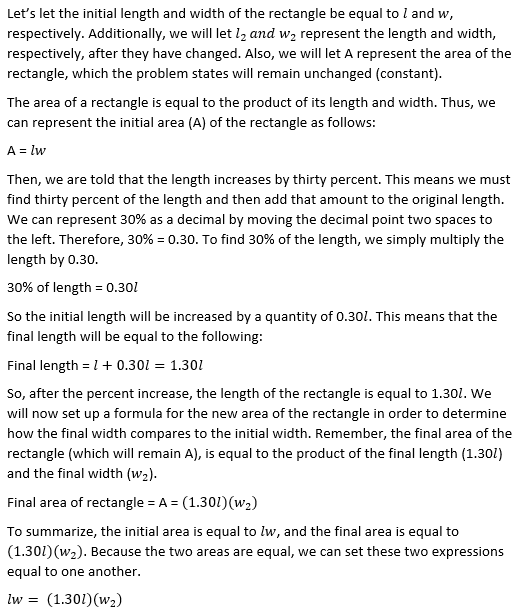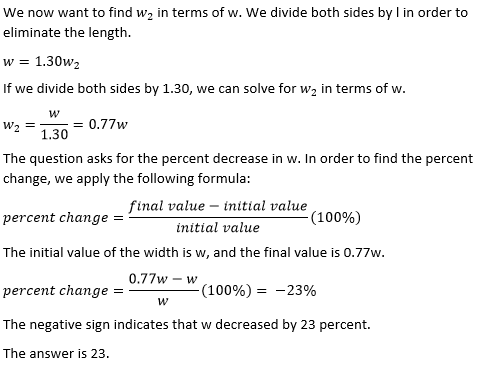### Example Question #11 : Percent Of Change

If the price of a TV was decreased from $3,000 to$1,800, by what percent was the price decreased?

60%

40%

30%

50%

20%

40%

Explanation:

The price was lowered by $1,200 which is 40% of$3,000.

### Example Question #1 : How To Find The Percent Of Decrease

If a rectangle's length decreases by fifteen percent, and its width decreases by twenty percent, then by what percent does the rectangle's area decrease?

45

36

35

40

32

32

Explanation:

Let's call the original length and width of the rectangle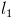and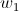, respectively.

The initial area,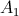, of the rectangle is equal to the product of the length and the width. We can represent this with the following equation: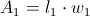Next, let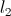and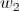represent the length and width, respectively, after they have been decreased.  The final area will be equal to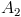, which will be equal to the product of the final length and width.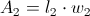We are asked to find the change in the area, which essentially means we want to compareand. In order to do this, we will need to find an expression forin terms ofand. We can rewriteandin terms ofand.

First, we are told that the length is decreased by fifteen percent. We can think of the full length as 100% of the length. If we take away fifteen percent, we are left with 100 – 15, or 85% of the length. In other words, the final length is 85% of the original length. We can represent 85% as a decimal by moving the decimal two places to the left.= 85% of=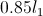Similarly, if we decrease the width by 20%, we are only left with 80% of the width.= 80% of=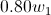We can now express the final area in terms ofandby substituting the expressions we just found for the final length and width.= ()() =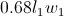Lastly, let's apply the formula for percent of change, which will equal the change in the area divided by the original area. The change in the area is equal to the final area minus the original area.

percent change =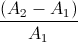(100%)

=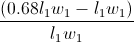(100%)

=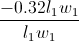(100%) = –0.32(100%) = –32%

The negative sign indicates that the rectangle's area decreased. The change in the area was a decrease of 32%.

### Example Question #1 : How To Find The Percent Of Decrease

The cost of a load of laundry is reduced by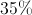. The cost is then reduced 2 weeks later by another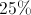. What is the overall reduction?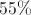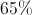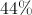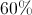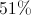Explanation:

The original reduction brings the total toof the original value. Taking adiscount off that price gives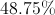of the original value. This means the reduction had been.

### Example Question #12 : Percent Of Change

The cost of a shirt in January isdollars. In February, the cost is decreased by 10%. In March, the cost is decreased by another 10%. By what percentage did the shirt decrease in total between January and March?We must know the original cost to find the answer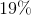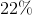Explanation:

The best way to answer this question is to plug in a number for n. Since you are working with percentages, it may be easiest to use 100 for n.

We know that in the month of February, the cost of this shirt was decreased by 10%. Because 10% of 100 is $10, the new cost of the shirt is$90.

In March, the cost of the shirt decreased another 10%. 10% of 90 is 9, so the cost of the shirt is now $81. To find the total percentage decrease, you must divide 81 by 100 and subtract it from 1. 1 – (81/100) = 1 – 0.81 = 0.19 The total decrease was 19%. ### Example Question #91 : Percentage The cost of a hat increases by 15% and then decreases by 35%. After the two price changes, the new price of the hat is what percent of the original? Possible Answers: 75% 85.3% 74.75% 80% 88.91% Correct answer: 74.75% Explanation: The easiest way to do percentage changes is to keep them all in one equation. Therefore, we would say that an increase of 15% is the same as multiplying the original value by 1.15. Likewise, we would say that a discount by 35% is the same as multiplying the original by .65. For our problem, let the hat cost X dollars originally. Therefore, after its increase, it costs 1.15X dollars. Now, we can consider this new price as the whole to which the discount will be applied. Therefore, a 35% reduction is (1.15X) * 0.65. Simplifying, we get 0.7475, or 74.75%. ### Example Question #2 : How To Find The Percent Of Decrease A dress is reduced in price by, but it still doesn't sell, so the manager discounts it by another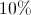. What is the total percentage discount? Possible Answers: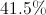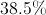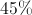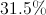None of the other answer choices are correct. Correct answer:Explanation: For these type of questions, it is always best to pretend that we are beginning with a$100 item and to calculate from there.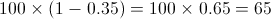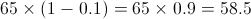If an item that is $100 is discounted by 35%, and then another 10%, the new price is 58.5%.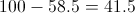The price difference (discount) is$41.5 for every \$100, or: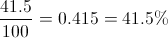The total discount is 41.5%.

### All PSAT Math Resources